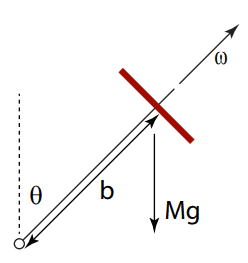# Precession of Rotating Rigid Body from Two Different Frames

## Homework Statement

A)
Use Euler's equations to solve for the precession rate of a rapidly spinning gyroscope precessing uniformly (no nutation). Use I3 for the moment along the z axis along the axle. Beginning with Euler's equations, derive the coupled equations:
## \dot ω_1 + Ω ω_2 = \frac {N_1} {I_1} ## where ## Ω = \frac {I_3 - I_1} {I_1} ω_3 ##
## \dot ω_2 + Ω ω_1 = \frac {N_2} {I_1} ##
And then solve for the precession rate of the gyroscope. (Remember the technique of adding i-times the second to the first equation, as for Focault’s pendulum.) Because Euler’s equations are in the body frame, the torque is time-varying with angular frequency ##ω_3##. Note that the gyroscope precession rate is very different than the force-free precession rate Ω.B) Solve the problem of 1) in the precessing frame. The precessing frame is neither the body frame or the fixed frame.

## Homework Equations

Euler's Equations:
$$N_1 = I_1\dot ω_1 - (I_2 - I_3) ω_2 ω_3$$
$$N_2 = I_2\dot ω_2 - (I_3 - I_1) ω_3 ω_1$$
$$N_3 = I_3\dot ω_3 - (I_1 - I_2) ω_1 ω_2$$

Angular Momentum:
L = Iω

Torque:
τ = r x F

## The Attempt at a Solution

A) At first I decided to break it down component by component...We can assume the rod is massless so we do not need to include the moment of the inertia of the rod. Setting the body coordinates to ##x_3## through the axle and out the top of the gyroscope parallel with the angular velocity the top is rotating with. ##x_2## is perpendicular to ##x_3## but in the same plane as the image pointing towards the top left of the image from the fixed point. ##x_1## is into the page. Here is an image with my frame of reference with ##x_3## being blue and ##x_2## being yellow.From there I have
$$I_1 = I_2$$
$$ω_1 = 0$$
$$ω_2 = \dot φ sinΘ$$
$$ω_3 = ω + \dot φ cosΘ$$
where ##\dot φ## is the rate of precession. I also have all of the time derivatives of the angular velocities are zero. Therefore, my Euler Equations are
##N_3 = 0##
##N_2 = 0##
##N_1 = -(I_1 - I_3)ω_2ω_3 = sinΘ*bmg##

However, in the given problem it wants the time derivative of the angular velocities to still be there and for ##N_2## to be nonzero. Are the time derivatives of the angular velocities nonzero because the entire frame is rotating with the body? I also still have ##ω_1## being zero which I don't know how it cannot be zero as there is no rotation in the x axis. I know that ##N_2## cannot be zero since there needs to be a toque being applied to balance out the torque being applied by the gravity so that ##N_2 = cosΘbmg##.

B)
For this one I'm not even sure if I am setting the frame correctly...My guess is to set the frame on the top of the gyroscope that is precessing and rotating. So I have the same convention as before just with the origin centered around the center of mass. Now I have all of the angular velocities being zero besides ##ω_3## since no other coordinate is rotating relative to this frame. Also all the time derivitives of the angular momentum are zero too since none of them are accelerating. However, when I do this I get zero applied torques which seems kind of right since I am in the frame of reference centered about where the torques would be...But I know the forces need to be balanced then and I don't know how I am going to balance the force due to gravity and I still need a force to cause myself to precess. I believe I am looking at this problem incorrectly but I just don't know where. I'm assuming it has something to do with my angular velocities or perhaps my rate of change of my angular velocities.